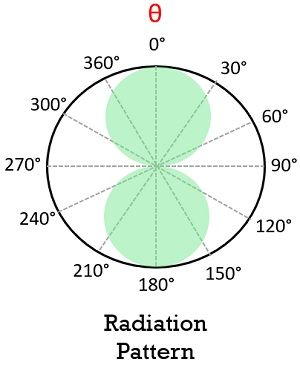# Half Wave Dipole Antenna

A type of antenna in which the length of the dipole is half the wavelength at the operating frequency is known as a half wave dipole antenna. It is considered as a very popular form of a dipole antenna and is sometimes known as Hertz antenna.

Generally, it is known to be the one with the simplest resonance structure in antennas for the transmission and receptions applications. It is present as the fundamental element of all the antenna shapes, as these are used for constructing various complex antennas.

The operating frequency range of the half wave dipole antennas lies between 3 kHz to 300 GHz.

## Content: Half Wave Dipole Antenna

### What is a Dipole Antenna?

We know that an electric dipole is defined as the separation of charges of opposite polarity (i.e., positive and negative). A dipole as an antenna is a horizontal metallic rod which is excited externally.

Generally, the dipole antenna is fed at the centre so as to transmit and receive RF energy.

It is considered to be as the simplest practical antenna. However, there is a classification of dipole antenna which is usually done on the basis of length of the electric dipole and its orientation.

Dipole antennas are generally classified as:

• Half Wave Dipole Antenna: It is an antenna having a length equal to half the wavelength at the frequency of operation.
• Quarter Wave Dipole Antenna: When the total length of the dipole is one-fourth of the wavelength at operating frequency then it is known as a quarter wave dipole.
• Folded Dipole Antenna: A folded dipole antenna is formed by combining two half wave dipole antennas in parallel orientation.
• Dual Dipole Antenna: Dual dipoles are the antennas that are formed by the combination of two dipoles and thus needs very large space.

Here we will discuss half wave dipole antenna.

### Construction

A half wave dipole antenna is an antenna constructed using a conductive tube or metallic element that has a length equal to the electrical half wavelength.

The excitation to the half wave dipole is provided at the centre. So, we can say that the feeding to the dipole is present at quarter wavelength point.

The figure below represents the structure of a half wave dipole antenna:Now, the question arises why specifically the antenna is fed at the centre and neither at the ends nor any other points?

So, basically while feeding an antenna, the major concern is related to the way of transferring the power from transmission line to the antenna with the least possible loss.

As there are two open ends of the conductor, thus voltage will be maximum but while moving towards the centre from the end voltage starts decreasing.

As against though the current was 0 at the ends along the length side of the radiating element, the current increases. Generally current reaches a maximum value at the centre which is 0 at the ends. In this way, there is sinusoidal variation in these quantities.

So, the external excitation to the antenna is provided at the centre, where the current is maximum and voltage is minimum. Due to this, there is minimal impedance to the feeder by the antenna.

## Working of Half Wave Dipole Antenna

We know that a half wave dipole is a metallic rod or thin wire having a physical length of half wavelength in free space. We have already discussed that excitation to this antenna is provided at the centre where the voltage is minimum while the current is maximum. Thus, the two quantities vary in a sinusoidal manner along the length of the radiating element.

So, as the voltage varies sinusoidally, so for the positive half of the voltage, when the amplitude is maximum then the charge carries inside the conductor gets attracted towards the positive applied potential.

As against, when the negative half of the sinusoidal signal is provided then the charges present in the conductor experience repulsion.

So, every time with the change in polarity of the applied signal, there is a change in the direction of flow of electrons inside the conducting material.

Hence, the back and forth movement of the electrons from an end to another causes it to get charged either positively or negatively.

This cumulative action of the electrons leads to generate current and the field is radiated from the half wave dipole.

As this antenna is a centre fed so, the maximum current offers maximum radiation in the plane which is in perpendicular direction. The radiation pattern of half wave dipole antenna is omnidirectional and is represented in the figure shown below:The advantages of half wave dipole antenna are as follows:

• These antennas do not show sensitivity to input impedance.
• The length of the antenna is appropriate for the free space characteristics with the operating frequency.
• The antenna is not bulky.
• It is cost-efficient.
• The input impedance of this dipole antenna properly matches with the impedance of the transmission line.